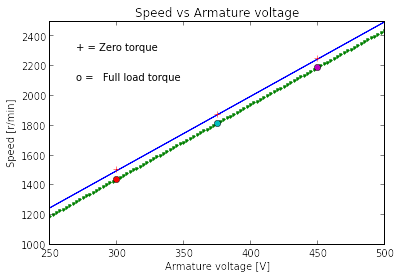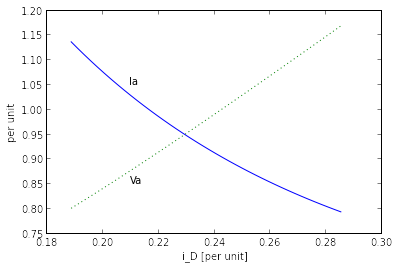# Chapter 11: Speed and Torque Control¶

### Example 11.1, Page number: 561¶

In :
from __future__ import division

#Variable declaration:
Vdc=240                             #DC supply (V)
D=0.75                              #Duty cycle
Rf=187                              #field resistance(ohm)
Lf=4.2                              #field winding inductance(H)
T=1                                 #switching period(msec)

#Calculations:
If=D*(Vdc/Rf)
tau=Lf/Rf                           #time constant(msec)
del_if=(2*Vdc/Rf)*(T/tau)*D*(1-D)

#Results:
print "Avg field current:",round(If,2),"A"
print "Magnitude of currnet ripple:",round(del_if,1),"mA"

Avg field current: 0.96 A
Magnitude of currnet ripple: 21.4 mA


### Example 11.2, Page number: 563¶

In :
from __future__ import division
from sympy import *
from math import *

#Variable declaration:
n1=1800                                 #r/min
n2=3600                                 #r/min
Va=240                                  #terminal voltage(V)
Ra=0.05                                 #Armature resistance(ohm)
Rsh=187                                 #Shunt field resistance(ohm)

#Calculations:
wm=symbols('wm')
wm1=float(2*pi*n1/60)
wm2=float(2*pi*n2/60)
return (22.4*(120*pi)**-3)*(wm)**3

Kf=Va/(Ifo*wm2)
def If(T,wm):
return (Va/(2*Kf*wm))*(1+sqrt(1-(4*wm*T*Ra)/Va**2))

Rf1tot=round(Va/float(If(T1,wm1)))
Rf2tot=round(Va/float(If(T2,wm2)))
Rrh1=Rf1tot-Rsh
Rrh2=Rf2tot-Rsh

#Results:
print "----------------------------------------------------------------"
print "r/min    Tload[N.m]      If[A]      R(f)tot[ohm]   Rrheostat[ohm]"
print "----------------------------------------------------------------"
print n1,"\t ",round(float(T1),1),"\t\t ",round(float(If(T1,wm1)),3),"\t",Rf1tot,"\t      ",Rrh1
print n2,"\t ",round(float(T2),1),"\t\t ",round(float(If(T2,wm2)),3),"\t",Rf2tot,"\t      ",Rrh2
print "----------------------------------------------------------------"

----------------------------------------------------------------
----------------------------------------------------------------
1800 	  14.9 		  0.678 	354.0 	       167.0
3600 	  59.4 		  0.333 	720.0 	       533.0
----------------------------------------------------------------


### Example 11.3, Page number: 567¶

In :
from __future__ import division
%matplotlib inline
from math import *
from pylab import *

#Variable declaration:
Rf=109                                  #Field resistance(ohm)
Vf=300                                  #Rated field voltage(V)
Ra=0.084                                #Armature resistance(ohm)

#Calculations:
If=Vf/Rf                                #Resulting field current(A)
P_rated=100*746                         #Watts
T_rated=P_rated/w_rated                 #Nm
Va=*102
for n in range(1,102,1):
Va[n-1]=250*(1+(n-1)/100)
T=0                                     #Zero torque
w=(Va[n-1]-T*Ra/(Kf*If))/(Kf*If)
T=T_rated
w=(Va[n-1]-T*Ra/(Kf*If))/(Kf*If)

print"The plot is as shown:"
plot (Va , NoLoadRPM , 'r+')
plot (Va , FullLoadRPM , ' o' )
plot (Va , FullLoadRPM ,'o' )
title('Speed vs Armature voltage')
xlabel('Armature voltage [V] ')
ylabel('Speed [r/min] ')
annotate('+ = Zero torque',xy=(270,2300))
ylim(1000,2500)
xlim(250,500)
show()

The plot is as shown:### Example 11.4, Page number: 571¶

In :
from __future__ import division
from sympy import *
from math import *

#Variable declaration:
Rf=109                                  #Field resistance(ohm)
Vf=300                                  #Rated field voltage(V)
n1=2000                                  #rpm
T_rated=285                            #Rated torque(Nm)
n2=1975                                 #Dropped rpm
Ra=0.084                                #Armature resistance(ohm)

#Calculations:
If=Vf/Rf                                #Resulting field current(A)
wm1=2*pi*n1/60
w_ref=wm1
Vao=Kf*If*wm1
Ia=T_rated/(Kf*If)
wm2=2*pi*n2/60
Ea=Kf*If*wm2
Va=Ea+Ia*Ra
G=symbols('G')
x=solve(Vao-round(Va)+G*(w_ref-wm2),G)

#Results:
print "Armature voltage,Vao:",round(Va,0),"V"

Armature voltage,Vao: 408.0 V


### Example 11.5, Page number: 573¶

In :
from __future__ import division
from math import *
from sympy import *

#Variable Declaration:
Ra=1.03                             #ohm
Va1=40                              #Armature voltage(V)
Va2=50                              #  "        "     "

#Calculations:
wm1=(Va1/(2*Km))*(1+sqrt(1-(4*Pl*Ra/Va1**2)))
wm2=(Va2/(2*Km))*(1+sqrt(1-(4*Pl*Ra/Va2**2)))

#Results:

for Va=40 V, wm= 169.2 rad/sec
for Va=50 V, wm= 217.5 rad/sec


### Example 11.6, Page number: 575¶

In :
from __future__ import division
from math import *

#Variable declaration:

Rf=109                                  #Field resistance(ohm)
Vf=300                                  #Rated field voltage(V)
Ra=0.084                                #Armature resistance(ohm)
#wm=2500                                 #rated r/min

#for part (1):
n1=2000                                 #r/min
n2=2500                                 #r/min

#Calculations:
#part (a):
If=Vf/Rf
w1=n1*2*pi/60
w2=n2*2*pi/60
Ea1=Kf*If*w1                    #Avg Amature voltage(V)
Ea2=Kf*If*w2
Ia1=n1*Tfl/(nf*Kf*If)
Ia2=n2*Tfl/(nf*Kf*If)
Va1 = Ea1 + Ia1*Ra
Va2 = Ea2 + Ia2*Ra
Tl1=(n1/nf)*Tfl
Tl2=(n2/nf)*Tfl

#part (b):

# The dynamic equation governing the speed of the motor is

#   wm=(pi/30)*n & wr=(pi/30)*nf
#   Tmech = Kf*If*Ia=Kf*If*(Va-Ea)/Ra          #Under armature-voltage control

#   Thus the governing differential equation is
#   d(wm)/dt + 48.4*wm - 24.7*Va = 0

#   wm = wf + (wi-wf)*exp(-t/tau)          #tau=1/48.4=20.7 msec
#   n = 2500- 50*exp( -t/tau )

#   The armature current will decrease exponentially with the
#   same 20.7 msec time constant from an initial value of
#   (Vf - Vi)/Ra = 1190 A to its final value of 149 A.

#   Ia = 149 + 1041*exp(-t/tau)

#part (c):
#        J*d(wm)/dt = Tmech-Tload = Tf-(Tf/wm)*wm
# or      d(wm)/dt + 1.18*wm - 310 = 0

#In this case, the speed will rise exponentially to wm=wf=262 rad/sec as
#         wm = 262-53*exp(-t/tau)           #tau=1/1.18=845 msec

#Results:
print "part(a):\n"
print "-------------------------------------------------"
print "-------------------------------------------------"
print n1,"\t",round(w1),"\t\t",round(Va1),"\t",round(Ia1),"\t",Tl1,"Nm"
print n2,"\t",round(w2),"\t\t",round(Va2),"\t",round(Ia2),"\t",Tl2,"Nm"
print "-------------------------------------------------"
print "\npart (b):"
print " The resultant motor speed, n = 2500 - 50*exp(-t/tau) where tau=20.7 msec"

print "\npart (c):"
print " The resultant motor speed, wm = 262 - 53*exp(-t/tau) where tau=845 msec"

part(a):

-------------------------------------------------
-------------------------------------------------
2000 	209.0 		410.0 	119.0 	228.0 Nm
2500 	262.0 		513.0 	149.0 	285.0 Nm
-------------------------------------------------

part (b):
The resultant motor speed, n = 2500 - 50*exp(-t/tau) where tau=20.7 msec

part (c):
The resultant motor speed, wm = 262 - 53*exp(-t/tau) where tau=845 msec


### Example 11.7, Page number: 581¶

In :
from __future__ import division
import math

#Variable Calculations:
f1=60                                #Initial frequency(Hz)
f2=50                               #Changed frequency(Hz)
Xs=0.836                            #Saturated synch reactance(ohm)
Va=1+0j                             #Armature voltage(V p.u)
Ia=1+0j                             #Armature current(A p.u)
If_rated=2.84                       #Rated field current(A)
p=6                                 #No. of poles

#Calculations:
#for part (a):
ns1=120*f1/p
ns2=120*f2/p
Eaf=Va-1j*Xs*Ia*exp(1j*0)          #field voltage(V)
Ifo=abs(Eaf)*If_rated                   #motor field current(A)

#for part(b):
#Eaf= (wm/wmo)*(If/Ifo)*Eafo
If=Ifo

#Results:
print "part(a):"
print "(i)  The motor speed:",ns1,"r/min"
print "(ii) The motor field current:",round(Ifo,2),"A"
print "part(b):"
print "(i)  The changed speed:",ns2,"A"
print "(ii) The mototr field current:",round(If,2),"A"

part(a):
(i)  The motor speed: 1200.0 r/min
(ii) The motor field current: 3.7 A
part(b):
(i)  The changed speed: 1000.0 A
(ii) The mototr field current: 3.7 A


### Example 11.8, Page number: 588¶

In :
from __future__ import division
from math import *
from sympy import *

#Variable declaration:
iF=2.84                                 #rated field current(A)
Vbase=220                               #base voltage(V)
Eaf=220/sqrt(3)                         #Rms voltage line-to-neutral(V)
f=60                                    #Hz
p=6                                     #poles
P_rated=45*10**3                        #rated power(W)
Xs_pu=0.836                             #per unit synchronous reactance(ohm)

#Calculations:
we=2*pi*f
Laf=sqrt(2)*Eaf/(we*iF)                 #Armature field reactance(H)
T_rated=P_rated/(we*2/p)
#setting rated values to reference values.
Tref=T_rated
iFref=iF
iQ=round((2/3)*(2/p)*Tref/(Laf*iFref),2)
iD=0

#since theta_me=wc*t, iD=0,we have,
t=symbols('t')
wc=120*pi
def ia(t):
return iD*cos(wc*t)-iQ*sin(wc*t)
def ib(t):
return iD*cos(wc*t-2*pi/3)-iQ*sin(wc*t-2*pi/3)
def ic(t):
return iD*cos(wc*t+2*pi/3)-iQ*sin(wc*t+2*pi/3)
Ibase=P_rated/(sqrt(3)*Eaf)
Imax=round(ia((pi/(2*wc))))
Ia=1j*abs(round(Imax/sqrt(2)))
Eaf=1j*we*Laf*iF/sqrt(2)
Zbase=Vbase**2/P_rated
Xs=Xs_pu*Zbase
Va=1j*Xs*Ia+Eaf                             #line-to-neutral voltage
Vt=abs(sqrt(3)*Va)/Vbase                #p.u terminal voltage(line-to-line)(V)

#Results:
print "part(a):"
print "\tia(t)=",ia(t),"A"
print "\tib(t)=",ib(t),"A"
print "\tic(t)=",ic(t),"A"
print "part(b):"
print "\tTerminal voltage:",round(float(Vt),2),"per unit"

part(a):
ia(t)= -167.01*sin(120*pi*t) A
ib(t)= 167.01*sin(120*pi*t + pi/3) A
ic(t)= -167.01*cos(120*pi*t + pi/6) A
part(b):
Terminal voltage: 1.3 per unit


### Example 11.9, Page number: 591¶

In :
from __future__ import division
from math import *

#Variable declaration:
iF=2.84                                 #rated field current(A)
Vrated=220                              #rated terminal voltage,l-l(V)
Ibase=118                               #base current(A)
Eaf=220/sqrt(3)                         #Rms voltage, line-to-neutral(V)
f=60                                    #Hz
p=6                                     #poles
P_rated=45*10**3                        #rated power(W)
Xs=0.899                                #Synchronous reactance(ohm)
Xs_pu=0.836                             #per unit synchronous reactance(ohm)
Tref=358                                #Reference torque(Nm) (from Ex11.8)

#Calculations:
Va=Vrated/sqrt(3)                        #base voltage, line to neutral(V)
we=2*pi*f
wm=(2/p)*we
Laf=sqrt(2)*Eaf/(we*iF)                 #Armature field reactance(H)
Ia=Tref*wm/(3*Va)
Ls=Xs/we                                #Synchronous inductance(mH)
delta=-atan(we*Ls*Ia/Va)
iQ_ref=sqrt(2)*Ia*cos(delta)
iD_ref=sqrt(2)*Ia*sin(delta)
iF_ref=(2./3)*(2/p)*Tref/(Laf*iQ_ref)

#since motor is running at rated voltage, base voltage and rated voltage
# are assumed to be same.
Va_pu=Va/Va
Ia_pu=Ia/Ibase

#Results:
print "The reqd motor field current:",round(iF_ref,2),"A"
print "Per unit voltage:",Va_pu,"p.u"
print "Per unit current:",round(Ia_pu),"p.u"

 The reqd motor field current: 3.7 A
Per unit voltage: 1.0 p.u
Per unit current: 1.0 p.u


### Example 11.10, Page number: 593¶

In :
from __future__ import division
from math import *

#Variable declaration:
ns=4000                             #rated speed(rpm)
Va=220                              #rated voltage(V)
Ls=1.75*10**-3                      #synchronous inductance(H)
Prated=25000                        #Watts
n=3200                              #rated OC speed(rpm)
p=2                                 #No. of poles

#Calculations:
#for part(a):
Eaf=Va/sqrt(3)
Trated=Prated/wm
we=(p/2)*n*pi/30
lambdaPM=sqrt(2)*Eaf/we             #flux linked wth permanent magnet(Wb)
Tref=Trated*0.65                #since motor is operated at 65% of Trated
iQref=(2./3)*(2/p)*(Tref/lambdaPM)

#for part(b:)
lambdaQ=Ls*iQref
lambdaa_base=Eaf/wm
lambda_pu=lambdaa/lambdaa_base

#for part(c)
Ia=sqrt((iDref**2+iQref**2)/2)          #rms armature current(A)
Ibase=Prated/(sqrt(3)*Va)
I_pu=Ia/Ibase

#Results:
print "(b)  Resultant armature flux linkage",round(lambda_pu,2),"p.u"
print "(c)  iD:",round(iDref,1),"A"
print "     Rms value of armature current:",round(Ia),"A"
print "     Per unit value of armature current:",round(I_pu,2),"A"

(a)  Required quadrature-axis current: 48.2 A
(b)  Resultant armature flux linkage 1.27 p.u
(c)  iD: -66.1 A
Rms value of armature current: 58.0 A
Per unit value of armature current: 0.88 A


### Example 11.11, Page number: 600¶

In :
from __future__ import division
from math import *

#Variable declaration:
V10=230/sqrt(3)
Nph=3
p=4
fe0=60
R1=0.095                                #Armature resistance(ohm)
R2=0.2                                  #Rotor resistance(ohm)
X10=0.680                               #Armature leakage reactance(ohm)
X20=0.672                               #Rotor leakage reactance(ohm)
Xm0=18.7                                #Inductice reactance(ohm)

#Calculations:
#taking two frequency values:
fe1=40
fe2=60

for m in range(1,3,1):
if m==1:
fe=fe1
else:
fe=fe2
X1=X10*(fe/fe0)
X2=X20*(fe/fe0)
Xm=Xm0*(fe/fe0)
V1=V10*(fe/fe0)

ws=4*pi*fe/p
ns=120*fe/p
V1eq=abs(V1*1j*Xm/(R1+1j*(X1+Xm)))
Z1eq=1j*Xm*(R1+1j*X1)/(R1+1j*(X1+Xm))
R1eq=Z1eq.real
X1eq=Z1eq.imag

#Search over the slip until the Pload = Pmech
s=0                             #slip initialised to 0
error=1

while error >=0:
s=s+0.00001
rpm=ns*(1-s)
wm=ws*(1-s)
Tmech=(1/ws)*Nph*V1eq**2*(R2/s)
Tmech = Tmech/((R1+R2/s)**2 + (X1+X2)**2)
Pmech=Tmech*wm

print "\nFor fe =",fe,"Hz :"
print "\tTerminal voltage=",round(V1*sqrt(3)),"V l-l"
print "\trpm =",round(rpm)
print "\tslip =",round(100*s,1),"%"

For fe = 40 Hz :
Terminal voltage= 153.0 V l-l
rpm = 1166.0
slip = 2.8 %

For fe = 60 Hz :
Terminal voltage= 230.0 V l-l
rpm = 1721.0
slip = 4.4 %


### Example 11.12, Page number: 608¶

In :
from __future__ import division
from math import *

#Variable declaration:
V10=230/sqrt(3)
Nph=3
p=4
fe0=60
R1=0.095                                #Armature resistance(ohm)
R2=0.2                                  #Rotor resistance(ohm)
X10=0.680                               #Armature leakage reactance(ohm)
X20=0.672                               #Rotor leakage reactance(ohm)
Xm0=18.7                                #Inductice reactance(ohm)
n=1680                                  #rpm
Pmech=9.7*10**3                         #Electromagnetic power(W)

#Calculations:
we0=2*pi*fe0
Lm=Xm0/we0
LS=Lm+X10/we0
LR=Lm+X20/we0
Ra=R1
RaR=R2
lambda_rated=sqrt(2)*V10/we0
#for specified operating condition
wm=n*(pi/30)
Tmech=Pmech/wm
Ia=sqrt((iD**2+iQ**2)/2)
wme=(p/2)*wm
we=wme+(RaR/LR)*(iQ/iD)
fe=we/(2*pi)
Va=sqrt(((Ra*iD-we*(LS-Lm**2/LR)*iQ)**2 + (Ra*iQ+we*LS*iD)**2)/2)

#Results:
print "Rms amplitude of the armature current:",round(Ia,1),"A"
print "The electrical frequency:",round(fe,1),"Hz"
print "Rms terminal voltage:",round(sqrt(3)*Va,1),"V  line-line"

Rms amplitude of the armature current: 27.9 A
The electrical frequency: 58.4 Hz
Rms terminal voltage: 243.6 V  line-line


### Example 11.13, Page number: 610¶

In :
from __future__ import division
%matplotlib inline
from math import *
from pylab import *

#Variable declaration:
P_rated = 12*10**3                       #Watts
V_rated = 230                            #Rated line-line voltage(v)
Va_rated = 230/sqrt(3)                   #Rated line to neutral(V)
fe_rated = 60                            #Hz
lambda_rated = sqrt(2)*Va_rated/we_rated #Wb
I_rated = P_rated/(sqrt(3)*V_rated)      #A
Ipeak_base = sqrt(2)*I_rated            #A
p = 4                                   #poles

V10=V_rated/sqrt(3)
R1=0.095                                #Armature resistance(ohm)
R2=0.2                                  #Rotor resistance(ohm)
X10=0.680                               #Armature leakage reactance(ohm)
X20=0.672                               #Rotor leakage reactance(ohm)
Xm0=18.7                                #Inductice reactance(ohm)

#Calculations:
Lm = Xm0/we_rated;
LS = Lm + X10/we_rated;
LR = Lm + X20/we_rated;
Ra = R1
RaR = R2
#operating point:
n = 1680                                #rpm
wm = n*pi/30
wme = (p/2)*wm
Pmech = 9.7*10**3
Tmech = Pmech/wm
lambda_DRpu=*42
iDpu=*42
Iapu=*42
fe=*42
Vapu=*42

for n in range(1,43,1):
iDpu[n-1]=iD/Ipeak_base
iQR=-(Lm/LR)**iQ
Ia=sqrt((iD**2+iQ**2)/2)
Iapu[n-1]=Ia/I_rated
we=wme-(RaR/LR)*(iQ/iD)
fe[n-1]=we/(2*pi)
Va_rms=sqrt(((Ra*iD-we*(LS-Lm**2/LR)*iQ)**2 +(Ra*iQ+ we*LS*iD)**2)/2)
Vapu[n-1]=Va_rms/Va_rated

#Results:
print "The required plot is as shown:"
plot(iDpu,Iapu)
plot(iDpu,Vapu,':')
xlabel('i_D [per unit] ')
ylabel('per unit')
annotate('Ia',xy=(0.21,1.05))
annotate('Va',xy=(0.21,0.85))
show()

The required plot is as shown: